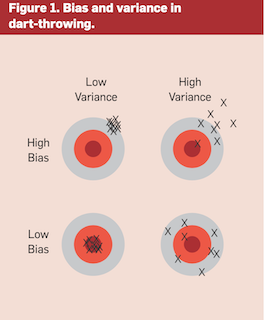Machine learning algorithms can figure out how to perform important tasks by generalizing from examples. This is often feasible and cost-effective where manual programming is not. As more data becomes available, more ambitious problems can be tackled.
Machine learning is widely used in computer science and other fields. However, developing successful machine learning applications requires a substantial amount of “black art” that is difficult to find in textbooks.
This article summarizes 12 key lessons that machine learning researchers and practitioners have learned. These include pitfalls to avoid, important issues to focus on, and answers to common questions.

## Gists 摘要

• Learning = Representation (表征) + Evaluation (评估) + optimization (优化)

Representation Evaluation Optimization
Instances Accuracy/Error rate Combinatorial optimization
K-nearest neighbor Precision and recall (精准率&召回率)   Greedy search (贪心搜索)
Support vector machines Squared error (平方误差)   Beam search (集束搜索)
Hyperplanes Likelihood (似然) Branch-and-bound (分支界限法)
Naive Bayes Posterior probability (后验概率) Continuous optimization
Logistic regression Information gain (信息增益)   Unconstrained
Decision trees K-L divergence (相对熵)     Gradient descent (梯度下降)
Sets of rules Cost/Utility     Conjugate gradient (共轭梯度)
Propositional rules Margin Quasi-Newton methods (拟牛顿法)
Logic programs Constrained
Neural networks   Linear programming (线性规划)
Bayesian networks (贝叶斯网络)
Conditional random fields (条件随机场)
• It’s Generalization that counts (泛化能力是ML的核心)
机器学习的基本目标是对训练集之外的样本进行泛化。

• Data alone is not enough (仅有数据是不够的)
先验知识

• Overfitting has many faces (过拟合具有多面性)

• 理解过拟合的一种方法是将泛化的误差进行分解，分为偏差和方差。

• bias可以理解是预测或估计很多次的均值
• variance表示很多次估计的方差
• 线性模型一般variance小，bias大
• 树模型一般variance大，bias小• 下面几个思路可能减小过拟合

• 交叉验证
• 加入正则项，避免模型过于复杂
• 没有噪声也会出现过拟合

• Intuition Fails in high Dimensions (直觉不适用于高纬度空间)

• 维数灾难
• 降维 (缺失值比率, 低方差滤波, 高相关滤波, 随机森林, PCA, t-SNE, UMAP…)
• Theoretical Guarantees are not What they seem (理论保证不一定可靠)

• Feature engineering is the Key
特征决定机器学习的上限，模型只是在逼近这个上限。

• More Data Beats a cleverer algorithm
收集更多的数据、处理更多的数据

• Learn many models, not Just one
bagging, boosting, stacking

• Simplicity Does not imply Accuracy (简单并不意味着准确)

• Representable Does not imply Learnable (可表示并不意味着可学习)

• Correlation Does not imply Causation (相关并不意味着因果)